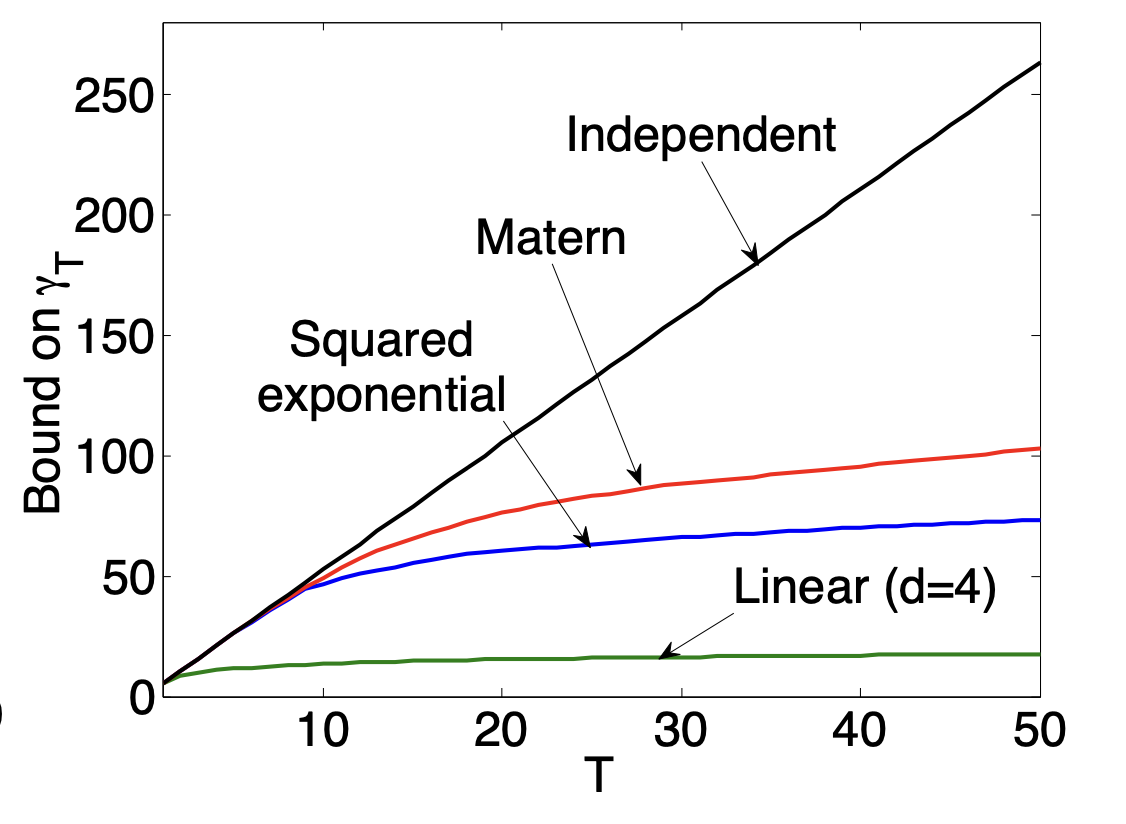# Gaussian Process Optimization in the Bandit Setting: No Regret and Experimental Design

July 25, 2023The authors resolve the important open problem of deriving regret bounds for this setting, which imply novel convergence rates for GP optimization.

We will discuss the following paper at 2pm:

Gaussian Process Optimization in the Bandit Setting: No Regret and Experimental Design

Many applications require optimizing an unknown, noisy function that is expensive to evaluate. We formalize this task as a multi-armed bandit problem, where the payoff function is either sampled from a Gaussian process (GP) or has low RKHS norm. We resolve the important open problem of deriving regret bounds for this setting, which imply novel convergence rates for GP optimization. We analyze GP-UCB, an intuitive upper-confidence based algorithm, and bound its cumulative regret in terms of maximal information gain, establishing a novel connection between GP optimization and experimental design. Moreover, by bounding the latter in terms of operator spectra, we obtain explicit sublinear regret bounds for many commonly used covariance functions. In some important cases, our bounds have surprisingly weak dependence on the dimensionality. In our experiments on real sensor data, GP-UCB compares favorably with other heuristical GP optimization approaches.

## Portfolio

Explore some of the materials optimised with Matterhorn.#### Photonite

Biosynthetic building material